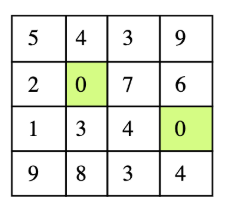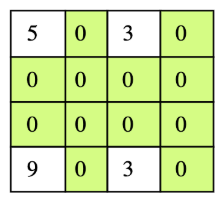# Make Columns and Rows Zero

## Problem Statement

Given a two-dimensional array, if any element within is zero, make its whole row and column zero. For example, consider the matrix below.There are two zeros in the input matrix at position (1,1) and (2,3). The output of this should be a matrix in which the first and second rows become zero and first and third columns become zeros. Below is the expected output matrix.## Hint

• Track rows / columns

## Try it yourself

void make_zeroes(vector<vector<int>>& matrix) {  //TODO: Write - Your - Code}

## Solution

void make_zeroes(vector<vector<int>>& matrix) {  if (matrix.empty()) {    return;  }  unordered_set<size_t> zero_rows;  unordered_set<size_t> zero_cols;  size_t rows = matrix.size();  size_t cols = matrix.size();  for (size_t i = 0; i < rows; ++i) {    for (size_t j = 0; j < cols; ++j) {      if (matrix[i][j] == 0) {        if (zero_rows.find(i) == zero_rows.end()) {          zero_rows.insert(i);          }        if (zero_cols.find(j) == zero_cols.end()) {          zero_cols.insert(j);        }      }    }  }  for (size_t r : zero_rows) {    for (size_t c = 0; c < cols; ++c) {      matrix[r][c] = 0;    }  }  for (size_t c : zero_cols) {    for (size_t r = 0; r < rows; ++r) {      matrix[r][c] = 0;    }  }}bool is_row_or_col_zero(vector<vector<int>>& matrix, int r, int c) {  size_t rows = matrix.size();  size_t cols = 0;  if (rows > 0) {    cols = matrix.size();  }  for (int i = 0; i < cols; ++i) {    if (matrix[r][i] == 0) {      return true;    }  }  for(int i = 0; i < rows; ++i) {    if (matrix[i][c] == 0) {      return true;    }  }  return false;}void verify(vector<vector<int>>& matrix) {  auto mat_copy = matrix;  make_zeroes(matrix);  size_t rows = matrix.size();  size_t cols = 0;  if (rows > 0) {    cols = matrix.size();  }  for (int i = 0; i < rows; ++i) {    for (int j = 0; j < cols; ++j) {      if (is_row_or_col_zero(mat_copy, i, j)) {        assert(matrix[i][j] == 0);      }    }   }}int main(int argc, char const *argv[]){  vector<vector<int>> matrix = {    {1, 5, 45, 0, 81},    {6, 7, 2, 82, 8},    {20, 22, 49, 5, 5},    {0, 23, 50, 0, 92}  };    print_matrix(matrix);  verify(matrix);  print_matrix(matrix);  matrix = create_random_matrix(5, 5);  print_matrix(matrix);  verify(matrix);  print_matrix(matrix);  for (int i = 0; i < 25; i++) {    for (int j = 0; j < 25; j++) {      matrix = create_random_matrix(i, j);      verify(matrix);    }  }  return 0;}

## Solution Explanation

### Runtime Complexity

O(m.n) where m is number of rows and n is number of columns.

O(m + n).

### Solution Breakdown

One naive solution which may come to the mind is to do a scan of the 2D matrix and upon seeing a zero, make that whole column and row zero. But that is not correct; even if there is only 1 zero in the matrix, this will make the whole matrix zero.

Instead, do a scan of the 2D array and identify all the rows and columns that have a zero in them and store them in two sets.

For the above example, set ‘zero_rows’ consists of two elements, 1 and 2 because there is a zero in row 1 and row 2.

Similarly, set ‘zero_columns’ consists of two elements, 1 and 3 because there is a zero in column 1 and column 3.

Practice problems like this and many more by checking out our Grokking the Coding Interview: Patterns for Coding Questions course!

Learn in-demand tech skills in half the time